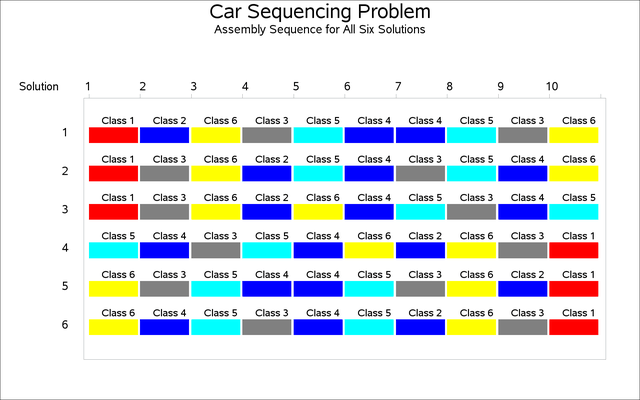### Example 3.7 Car Sequencing Problem

This problem is an instance of a category of problems known as the car sequencing problem. There is a considerable amount of literature related to this problem (Dincbas, Simonis, and Van Hentenryck, 1988; Gravel, Gagne, and Price, 2005; Solnon et al., 2008).

A number of cars are to be produced on an assembly line where each car is customized with a specific set of options such as air-conditioning, sunroof, navigation, and so on. The assembly line moves through several workstations for installation of these options. The cars cannot be positioned randomly since each of these workstations have limited capacity and need time to set up the options as the assembly line is moving in front of the station. These capacity constraints are formalized using constraints of the formout of
, which indicates that the workstation can install the option onout of every sequence ofcars. The car sequencing problem is to determine a sequencing of the cars on the assembly line that satisfies the demand constraints for each set of car options and the capacity constraints for each workstation.

This example comes from Dincbas, Simonis, and Van Hentenryck (1988). Ten cars need to be customized with five possible options. A class of car is defined by a specific set of options; there are six classes of cars.

The instance data are presented in Table 3.10.

Table 3.10: The Instance Data

Option

Capacity

Car Class

Name

Type

m/N

1

2

3

4

5

6

Option 1

1

1/2

1

0

0

0

1

1

Option 2

2

2/3

0

0

1

1

0

1

Option 3

3

1/3

1

0

0

0

1

0

Option 4

4

2/5

1

1

0

1

0

0

Option 5

5

1/5

0

0

1

0

0

0

Number of Cars

1

1

2

2

2

2

For example, car class 4 requires installation of option 2 and option 4, and two cars of this class are required. The workstation for option 2 can process only two out of every sequence of three cars. The workstation for option 4 has even less capacity—two out of every five cars.

The instance data for this problem is used to create a SAS data set, which in turn is processed to generate the SAS macro variables shown in Table 3.11 that are used in the CLP procedure. The assembly line is treated as a sequence of slots, and each car must be allocated to a single slot.

Table 3.11: SAS Macro Variables

Macro Variable

Description

Value

Ncars

Number of cars (slots)

10

Nops

Number of options

5

Nclss

Number of classes

6

Max_1–Max_5

For each option, the maximum number of cars with that option in a block

1 2 1 2 1

Blsz_1–Blsz_5

For each option, the block size to which the maximum number refers

2 3 3 5 5

class_1–class_6

Index number of each class

1 2 3 4 5 6

cars_cls_1–cars_cls_6

Number of cars in each class

1 1 2 2 2 2

list_1–list_5

Class indicator list for each option; for example, classes 1, 5, and 6 that require option 1 (list_1)

list_1=1,0,0,0,1,1 list_2=0,0,1,1,0,1 list_3=1,0,0,0,1,0 list_4=1,1,0,1,0,0 list_5=0,0,1,0,0,0

cars_opts_1–cars_opts_5

Number of cars for each option (cars_opts_1 represents the number of cars that require option 1)

5 6 3 4 2

The decision variables for this problem are shown in Table 3.12.

Table 3.12: The Decision Variables

Variable Definition

Description

S_1–S_10=[1,6]

S_is the class of cars assigned to slot.

O_1_1–O_1_5=[0,1] … O_10_1–O_10_5=[0,1]

O__=1 if the class assigned to slotneeds option.

In the following SAS statements, the workstation capacity constraints are expressed using a set of linear constraints for each workstation. The demand constraints for each car class are expressed using a single GCC constraint. The relationships between slot variables and option variables are expressed using an element constraint for each option variable. Finally, a set of redundant constraints are introduced to improve the efficiency of propagation. The idea behind the redundant constraint is the following realization: if the workstation for optionhas capacityout of, then at mostcars in the sequence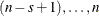can have optionwhereis the total number of cars. Consequently at least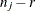cars in the sequence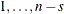must have option, where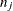is the number of cars with option. Generalizing this further, at least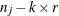cars in the sequence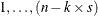must have option,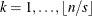.


%macro car_sequencing(outdata);

proc clp out=&outdata varselect=minrmaxc findall;

/* Declare Variables */
var
/* Slot variables: Si - class of car assigned to Slot i */
%do i = 1 %to &Ncars;
S_&i = [1, &Nclss]
%end;

/* Option variables: Oij
- indicates if class assigned to Sloti needs Option j */
%do i = 1 %to &Ncars;
%do j = 1 %to &Nops;
O_&i._&j = [0, 1]
%end;
%end;
;

/* Capacity Constraints: for each option j */
/* Installed in at most Max_j cars out of every sequence of BlSz_j cars */
%do j = 1 %to &Nops;
%do i = 0 %to %eval(&Ncars-&&BlSz_&j);
lincon 0
%do k=1 %to &&BlSz_&j;
+ O_%eval(&i+&k)_&j
%end;
<=&&Max_&j;
%end;
%end;

/* Demand Constraints: for each class i */
/* Exactly cars_cls_i cars */
gcc (S_1-S_&Ncars) = (
%do i = 1 %to &Nclss;
(&&class_&i, &&cars_cls_&i, &&cars_cls_&i)
%end;
);

/* Element Constraints: For each slot i and each option j */
/* relate the slot variable to the option variables.      */
/*  O_i_j is the S_i th element in list_j.                */
%do i = 1 %to &Ncars;
%do j = 1 %to &Nops;
element (S_&i, (&&list_&j), O_&i._&j);
%end;
%end;

/* Redundant Constraints to improve efficiency - for every  */
/*    option j.                                             */
/* At most &&Max_&j out of every sequence of &&BlSz_&j cars */
/*    requires option j.                                    */
/* All the other slots contain at least cars_opt_j - Max_j  */
/*    cars with option j                                    */
%do j = 1 %to &Nops;
%do i = 1 %to %eval(&Ncars/&&BlSz_&j);
lincon 0
%do k=1 %to %eval(&Ncars-&i*&&BlSz_&j);
+ O_&k._&j
%end;
>= %eval(&&cars_opts_&j-&i*&&Max_&j);
%end;
%end;
run;

%mend;
%car_sequencing(sequence_out);



This problem has six solutions, as shown in Output 3.7.1.

Output 3.7.1: Car Sequencing